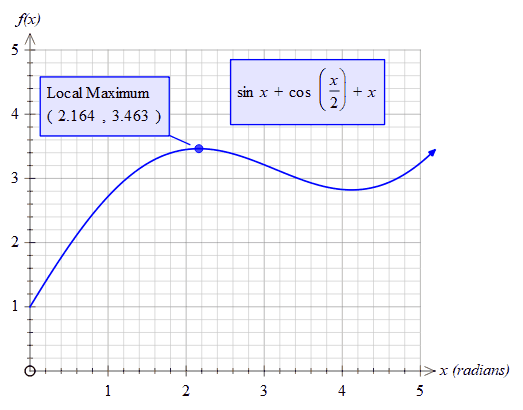﻿ fnMax

# fnMax

Top  Previous  Next
 fnMax(function, low, high)   Finds the x value which produces the highest y value between low and high. For example:fnmax(sinx+cos(x/2)+x,1,3) = 2.164 fnmax(sinx+cos(x/2)+x,0,1) = 1   To find the y value of the local maximum, use the fnmaxval function